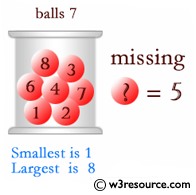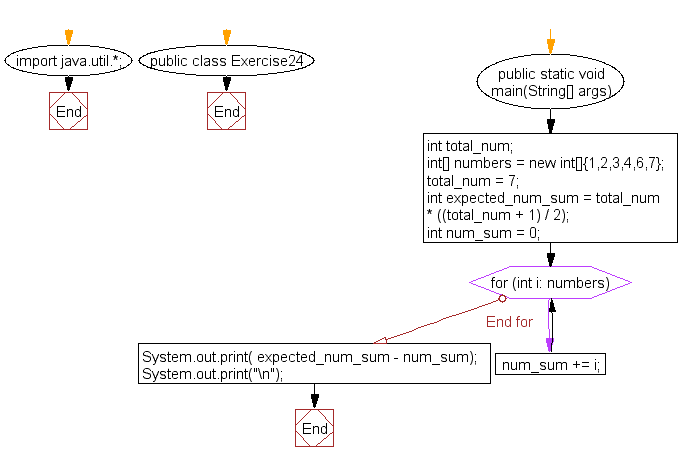﻿ Java exercises: Find a missing number in an array - w3resource# Java Array Exercises: Find a missing number in an array

## Java Array: Exercise-24 with Solution

Write a Java program to find a missing number in an array.

Pictorial Presentation:Sample Solution:

Java Code:

``````import java.util.*;
public class Exercise24 {
public static void main(String[] args) {

int total_num;
int[] numbers = new int[]{1,2,3,4,6,7};
total_num = 7;
int expected_num_sum = total_num * ((total_num + 1) / 2);
int num_sum = 0;
for (int i: numbers) {
num_sum += i;
}
System.out.print( expected_num_sum - num_sum);
System.out.print("\n");
}
}
```
```

Sample Data: 1,2,3,4,6,7

Sample Output:

```
5
```

Flowchart:Visualize Java code execution (Python Tutor):

Java Code Editor:

Improve this sample solution and post your code through Disqus

What is the difficulty level of this exercise?

Test your Programming skills with w3resource's quiz.

﻿

## Java: Tips of the Day

countOccurrences

Counts the occurrences of a value in an array.

Use Arrays.stream().filter().count() to count total number of values that equals the specified value.

```public static long countOccurrences(int[] numbers, int value) {
return Arrays.stream(numbers)
.filter(number -> number == value)
.count();
}
```

Ref: https://bit.ly/3kCAgLb